# Gas Dynamics

Also found in: Wikipedia.

## Gas dynamics

The study of gases in motion. In general, matter exists in any of three states: solid, liquid, or gas. Liquids are incompressible under normal conditions; water is a typical example. In contrast, gases are compressible fluids; that is, their density varies depending on the pressure and temperature. The air surrounding a high-speed aircraft is an example.

Gas dynamics can be treated in a variety of ways. One such way deals with gases as a continuum. The structure of gases on the particle level is called rarefied gas dynamics. See Aerothermodynamics, Compressible flow, Fluid flow

Gases in motion are subject to certain fundamental laws. These are the laws of the conservation of mass, momentum, and energy. In the case of the dynamics of incompressible fluids, it is usually sufficient to satisfy only the laws of conservation of mass and momentum. This distinction constitutes the fundamental difference between high-speed gas dynamics and hydrodynamics. If irreversibilities are involved, a fourth equation called the entropy balance equation may be considered. Whereas mass, momentum, and energy are conserved, the entropy is not. Real problems are irreversible; that is, losses such as friction are involved. However, as a first approximation such effects are generally not considered. See Conservation laws (physics), Conservation of energy, Conservation of mass, Conservation of momentum

The mass, momentum, and energy equations are higher-order, nonlinear equations that have no general solution, and only a limited number of special cases can be solved. Another approach is to resort to numerical solutions using high-speed digital computers. While this approach has proven to be very useful, it limits the degree to which flow phenomena can be conceptualized. Accordingly, it is frequently permissible to write the equations in one-dimensional form. By one-dimensional flow is meant that the properties of gas such as its velocity and density are assumed to be constant in the direction perpendicular to the direction of the gas flow. Generally, the one-dimensional approach gives excellent insights into understanding the physical behavior of the flow. It is also very useful in setting up the computer algorithm for numerical solutions. See Computational fluid dynamics

One other matter must be considered, namely whether the flow is steady or unsteady. In steady flow, the flow characteristics do not vary with time, whereas unsteady flow implies that the flow assumes different configurations over time. Thus, unsteady flow is broader in scope. In this case the continuity equations for conservation of mass may be written as Eq. (1).

(1)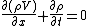In this equation, the first term defines the mass-flow changes with respect to the space coordinates, whereas the second term indicates the changes with time. Here, ∂ is the partial differential operator; x denotes the space coordinate, in this case the direction of flow; &rgr; is the gas density; V is the gas velocity; and t is the time.

If the flow is steady, there is no time-dependent term, and hence the continuity equation can be written in integrated form as Eq. (2), where A denotes the area in the direction perpendicular to the flow direction.

(2)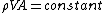The momentum equation is the mathematical representation of the law of conservation of momentum. It is a statement of the forces acting on the gas. Different types of forces must be recognized. Body forces, such as gravitation and electromagnetic forces, act at a distance. The so-called surface forces may assume different forms, such as normal stresses and viscosity. The simple form of the momentum equation is Eq. (3),

(3)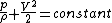which, in spite of its simplicity, is very powerful. Called Bernoulli's theorem, this equation makes a crucial statement that when the velocity increases, the pressure p decreases. See Bernoulli's theorem

The energy equation expresses the first law of thermodynamics and accounts for the changes in energy as the gas moves about its path. It can also take into consideration energy exchanges between the gas and its environment, such as radiation. Its simplest form is Eq. (4), where cp denotes the specific heat at constant pressure.

(4)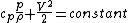The speed of sound or the acoustic velocity is a very important term in gas dynamics because it serves as a criterion to identify flow regimes. Being able to do so is crucial because the designer must know the conditions that the gas will generate or, conversely, experience. In prescribing flow regimes, the flow velocity of the gas is compared with the acoustic velocity. This ratio, called the Mach number (M), is defined by Eq. (5). (5)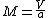Using the Mach number the following flow regimes are described: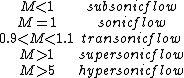High-speed aircraft are categorized by the Mach number. See Mach number, Sound

Flows can be classified as internal flow and external flow. Internal flow refers to the cases where the gas is constrained by a duct of some sort. Characteristically external flow is flow over an airplane or missile. Internal flows are conveniently characterized by (1) the shape of the duct and its variation, (2) the heat transfer through the walls of the duct and internal heat sources, and (3) frictional effects. By varying one of these characteristics at a time, the essential features of internal flow can be discussed most simply.

Boundary layers and wakes are the centers of interest in external flows. Here the effects of compressibility are substantially more difficult to analyze than in internal flows, if for no other reason than the inapplicability of a one-dimensional approach. See Boundary-layer flow, Wake flow

Rarefied gas dynamics is that branch of gas dynamics dealing with the flow of gases under conditions where the molecular mean free path is not negligibly small compared to some characteristic dimension of the flow field. Rarefied flows occur when the gas density is extremely low, as in the cases of vacuum systems and high-altitude flight, but also when gases are at normal densities if the characteristic dimension is sufficiently small, as in the case of very small particles suspended in the atmosphere.

The dimensionless parameter which describes the degree of rarefaction existing in a flow is the Knudsen number, Kn = λ/L, defined as the ratio of the mean free path λ to some characteristic dimension L of the flow field. Depending on the situation, L might be chosen, for example, as the diameter of a duct in a vacuum system, the wavelength of a high-frequency sound wave, the diameter of a suspended submicrometer-size particle, the length of a high-altitude rocket, or the thickness of a boundary layer or a shock wave. The mean free path λ, which is the average distance traveled by a gas molecule between successive collisions with other molecules, is equal to the molecular mean speed, given by Eq. (6)

(6)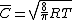[where R is the gas constant and T is the gas temperature], divided by the collision frequency &ngr;c: thus, Eq. (7)
(7)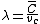is satisfied. However, it is often more convenient in evaluating the Knudsen number to use the viscosity-based mean free path given by Eq. (8), where &ngr; is the kinematic viscosity.
(8)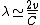See Viscosity

It is convenient to divide rarefied flows into three flow regimes, according to the range of values of the appropriate Knudsen numbers. The regime of highly rarefied flow, which obtains for Kn much greater than 1 (typically greater than 10), is called collisionless or free-molecule flow, while the regime of slight rarefaction, where Kn is much less than 1 (typically less than 0.1), is called slip flow. Flows at Knudsen numbers intermediate to these limiting values are termed transition flows. The phenomena and methods of analysis associated with the three regimes are in general quite dissimilar.

McGraw-Hill Concise Encyclopedia of Physics. © 2002 by The McGraw-Hill Companies, Inc.
The following article is from The Great Soviet Encyclopedia (1979). It might be outdated or ideologically biased.

## Gas Dynamics

the branch of hydroaeromechanics that deals with the movement of compressible gaseous and liquid mediums and their interactions with solids. As a part of physics, gas dynamics is related to thermodynamics and acoustics.

The property of compressibility is the ability of a substance to change its original volume under the influence of a pressure differential or change in temperature. Therefore, compressibility becomes essential only at speeds of movement comparable to the speed of sound in a given medium, or when large pressure differentials or temperature gradients form in the medium. Modern gas dynamics also deals with the flow of gases at the high temperatures that are accompanied by chemical processes (dissociation, combustion, and other chemical reactions) and physical processes (ionization or radiation). The study of the movement of gases under conditions in which the gas cannot be considered a continuous medium (when the interaction with each other and with solid bodies of the molecules composing it must be examined) belongs to the field of aerodynamics of rarefied gases, which is based on the kinetic molecular theory of gases. The dynamics of a compressible gas at low speeds of movement of large air masses in the atmosphere constitutes the basis of dynamic meteorology. Gas dynamics took shape historically as a further development and generalization of aerodynamics; therefore they are often considered as a single science—“air-gas dynamics.”

The theoretical foundation of gas dynamics is formed by the application of the basic laws of mechanics and thermodynamics to a moving volume of compressible gas. The Navier-Stokes equations, which describe the movement of a viscous, compressible gas, were developed in the first half of the 19th century. The German scientist B. Riemann (1860), the English scientist W. Rankine (1870), and the French scientist H. Hugoniot (1887) investigated the propagation of shock waves in a gas. These waves appear only in compressible mediums, and their speed is greater than the speed of sound in the mediums. Riemann also developed the foundations of the theory of unsteady motion of a gas—that is, motion for which the parameters of gas flow at every point change with time.

S. A. Chaplygin’s work On Gas Streams, published in 1902, played a fundamental part in the formation of gas dynamics as an independent science. Chaplygin’s methods of solving problems of gas dynamics became widespread. In 1908 the German scientists L. Prandtl and T. Mayer, who investigated a special case of gas flow with continuous acceleration, discovered a fruitful method of solving problems of gas dynamics. In 1922 the Soviet scientist A. A. Fridman laid the foundations of dynamic meteorology in his work An Experiment on the Hydromechanics of a Compressible Liquid. In 1929 the German scientists L. Prandtl and A. Busemann developed an effective graph-numerical method of solving a wide range of problems of gas dynamics, which was applied in 1934 by the Soviet scientist F. I. Frankl’ to more complex cases of gas currents. These methods are widely used when problems of gas dynamics are solved with the aid of computers. A gas dynamics laboratory was first created in the USSR in 1921; in 1927 it was reorganized as a research institute. This institute worked together with the Group for the Study of Jet Propulsion (1932) in setting the foundations for Soviet rocketry.

Gas dynamics has existed as an independent branch of hydroaeromechanics since 1930, when the increasing speeds in aviation necessitated the serious study of the compressibility factor in studying the movement of air. The first International Congress on Gas Dynamics was convened in Rome in 1935. The intensive development of gas dynamics began during and after World War II in connection with the wide use of gas dynamics in technology: jet aviation, rocket weaponry, rocket and jet engines; supersonic aircraft and missiles; and the creation of nuclear bombs, whose explosion creates powerful blast and shock waves. In this period of the development of gas dynamics, major contributions were made by such researchers as the Soviet scientists S. A. Khristianovich. A. A. Dorodnitsyn, L. I. Sedov, G. I. Petrov, and G. G. Chernyi; the German scientists Prandtl and Busemann; the English scientists G. Taylor and J. J. Lighthill; the American scientists T. von Kármán, A. Ferri, and W. Hayes; the Chinese scientist Ch’ien Hsüeh-sen; and scientists from other countries.

In designing various apparatus, engines, and gas-powered vehicles, the task of gas dynamics is to define the forces of pressure and friction and of temperature and heat flow at any point along the surface of a body or duct that is in contact with gas at any moment. In studying the propagation of gas jets, blast and shock waves, or combustion and detonation according to the methods of gas dynamics, the pressure, temperature, and other parameters of the gas are determined over the whole field of propagation. The study of the difficult tasks posed by technology has made modern gas dynamics into a science dealing with the motion of arbitrary gas mixtures, which may contain solid and liquid particles as well—for example, the exhaust gases of rocket engines operating on liquid or solid fuel. In addition, the parameters that characterize the state of these gases (pressure, temperature, density, electrical conductivity, and others) may vary widely.

The development of modern gas dynamics is characterized by a close association of theoretical methods, the use of electronic computers, and complicated aerodynamic and physical experiments. Theoretical representations, which may rely in part on experimental data, make it possible to describe in equations the motion of gas mixtures of complex composition, including multiphase mixtures, during physicochemical transformations. Applied mathematics is being used to develop efficient ways of solving these equations on computers. Finally, experimental data are being used to determine the necessary order of magnitude of the physical and chemical features inherent in the medium and processes under investigation (the coefficients of viscosity and thermal conductivity, the rate of chemical reactions, relaxation times, and so on).

Many problems raised by modern technology cannot yet be solved theoretically by gas dynamics. In these cases a solution is found experimentally, based on similarity theory and the laws of hydrodynamic and aerodynamic simulation. Gas dynamics experiments are carried out in air and gas dynamics laboratories in supersonic and hypersonic wind tunnels, ballistics units, shock tubes, pulse tubes, and other specialized gas-dynamics units.

The laws of gas dynamics are widely applied in exterior and interior ballistics and in the study of such phenomena as explosion, combustion, detonation, and condensation in a moving current. Simplified theoretical representations of the parameters of a gas stream averaged over the cross section, as well as the main principles of motion (found experimentally), are widely used in applied gas dynamics in the design of compressors, turbines, nozzles, diffusers, rocket motors, wind tunnels, ejectors, and gas pipelines.

Research in gas dynamics is carried out in the same institutes as research aerodynamics, and the results of these studies are published in the same journals and collections.

### REFERENCES

Osnovy gazovoi dinamiki. Edited by H. Emmons. Moscow, 1963. (Translated from English.)
Kármán, T. von. Sverkhzvukovaia aerodinamika: Printsipy i prilozheniia. Moscow, 1948. (Translated from English.)
Abramovich, G. N. Prikladnaia gazovaia dinamika, 3rd ed. Moscow, 1969.
Chernyi, G. G. Techeniia gaza s bol’shoi sverkhzvukovoi skorost’iu. Moscow, 1959.
Staniukovich, K. P. Neustanovivshiesia dvizheniia sploshnoi sredy. Moscow, 1955.
Zel’dovich, la. B., and Iu. P. Raizer. Fizika udarnykh voln i vysokotemperaturnykh gidrodinamicheskikh iavlenii, 2nd ed. Moscow, 1966.

S. L. VISHNEVETSKII

## gas dynamics

[¦gas dī′nam·iks]
(physics)
The study of the motion of gases, and of its causes, which takes into account thermal effects generated by the motion.
McGraw-Hill Dictionary of Scientific & Technical Terms, 6E, Copyright © 2003 by The McGraw-Hill Companies, Inc.
References in periodicals archive ?
The predictions of current models for gas dynamics in very faint active galaxies clearly failed."
Study researchers said that these observations failed predictions of&nbsp; current models for gas dynamics in very faint active galaxies.
The quasi-gasdynamic (QGD) system of equations [2, 3] is an entropy-correct and the Petrovsky parabolic [18, 21] regularization of the gas dynamics equations.
Hypersonic and High-Temperature Gas Dynamics, 3rd Edition
where [rho] [greater than or equal to] 0, u [greater than or equal to]0 are the density and the velocity, respectively, and p is the velocity offset which is called the "pressure" inspired from gas dynamics. The AR model was established to remedy the deficiencies of second order models of car traffic presented by Daganzo  and was independently obtained by Zhang .
 studied the failure law of coal via explosion of critical carbon dioxide and built a gas dynamics model.
Since then with the development of the computational methods for the solution of gas dynamics problems, the improvement of the kinetic schemes, and the growth of available computational resources, the continuous clarification of qualitative and quantitative characteristics of the process has occurred (for example, obtaining the three-dimensional spin mode of propagation, the thin structure of cellular detonation, and the detonation limits ).

Site: Follow: Share:
Open / Close